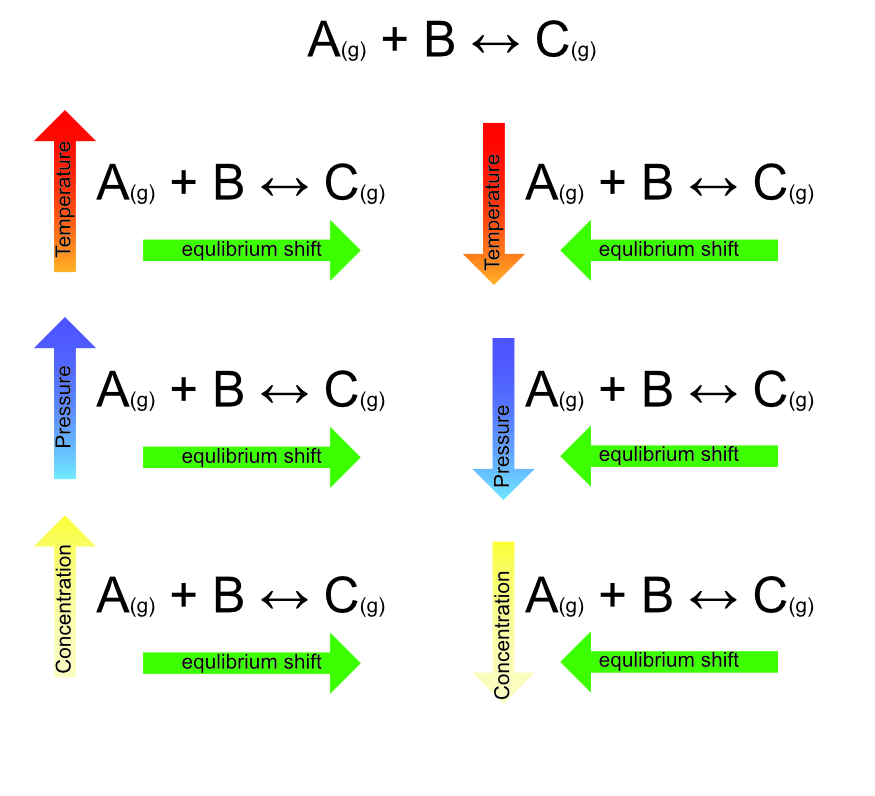# Equilibria

## Reversible Reactions

In chemical reactions the atoms in the reactants recombine to form the atoms and molecules of the products, taking in or giving out energy in the process. For most reactions, this change is permanent and cannot easily be undone. There are some reactions, however, where the reaction can be reversed. The direction of these reactions depends on the conditions around the reaction, including the relative concentration of reactants to products.

A normal reaction is written in the form:

A + B → C + D The “→” arrow indicates the change from reactants to products as a one-way process.

A reversible reaction using the symbol “⇌” to show that the reaction can flow in either direction.

``````    A + B ⇌ C + D
``````

The energy change for a reversible reaction is also reversed. If A + B → C + D is exothermic then C + D → A + B is endothermic.

Example

Heating of Ammonium chloride results in the production of ammonia and hydrochloric acid. However, adding ammonia to hydrochloric acid will result in the formation of ammonium chloride.

``````    NH4Cl(s) ⇌ NH3(g) + HCl(g)
``````

The forward reaction (left to right) is exothermic, the reverse reaction (right to left) is exothermic.

The reaction NH3(g) + HCl(g) → NH3Cl(s) will not reverse unless the ammonium chloride is heated, left at room temperature the ammonium chloride will not revert to its products.

## Dynamic Equilibrium

Some reversible reactions can flow in both directions at the same time. This means that they eventually reach a dynamic equilibrium. In this situation, the reaction is happening at the same rate in the forward direction as it is in the reverse direction. So although both reactions are still happening the overall concentration of the chemicals on both sides of the reaction remains constant.

If: A + B ⇌ C is a reversible reaction that can reach dynamic equilibrium the following are true:

1. A + B → C and C → A +B at the same time.
2. If the concentration of A and B are greater than C __then C is produced faster than it converts back to A+B. So, C_seems_ to be the product of the reaction,__the concentration of C increases.
3. If the concentration of C is greater than the concentration of A + B then A + B are produced faster than C. So A + B_seem_ to be the product of the reaction,the concentration of A + B increase.
4. If the concentration of A+B equals the concentration of C then A+B are being produced just as fast a C is being produced. There is no overall change in concentration of A, B or C. The process is in dynamic equilibrium.

Example - The Haber Process

Nitrogen + Hydrogen ⇌ Ammonia

N2 + 3H2 ⇌ 2NH3

The forward reaction (left to right) is exothermic, but the reverse reaction (right to left) is endothermic.

This reaction is known as the Haber process and is used for the production of ammonia that can then be converted into fertilizers. In order to ensure that the hydrogen and the nitrogen are converted to ammonia and to stop the process from reversing or reaching equilibrium, the ammonia is pumped away to stop the concentration increasing.

## Le Chatelier’s Principle

The direction in which a reversible reaction flows can be affected by:

1. Temperature
2. Pressure
3. Relative concentration of the chemicals.

For a reaction in dynamic equilibrium if these all remain constant so will the concentration of all the chemicals. However, if one of them is changed the concentrations will change to compensate for the change. This is known as Le Chatelier’s Principle.

If a dynamic equilibrium is disturbed by changing the conditions, the equilibrium moves to counteract the change.

A simple reversible reaction; A(g) + B(s) ⇌ C(g) is in equilibrium.

A + B → C is endothermic (takes in energy), and C → A + B is exothermic.

Changing Temperature

An increase in temperature means A + B gain energy and more bonds are broken (endothermic), therefore the equilibrium shifts to create more C.

Lowering the temperature releases energy from C to make more A + B.

Changing Pressure

Increasing the pressure of gas A will push the reaction to produce more of gas C until the pressures of A and C are again equal. Reducing the pressure of C would have the same effect as relative pressure between the gases is the important factor.

Reducing the pressure of gas A will reverse the reaction converting C to A +B until a new equilibrium is produced.

Changing Concentrations

The equilibrium will change to reduce the concentration of the highest side of the equation until a new equilibrium is reached.